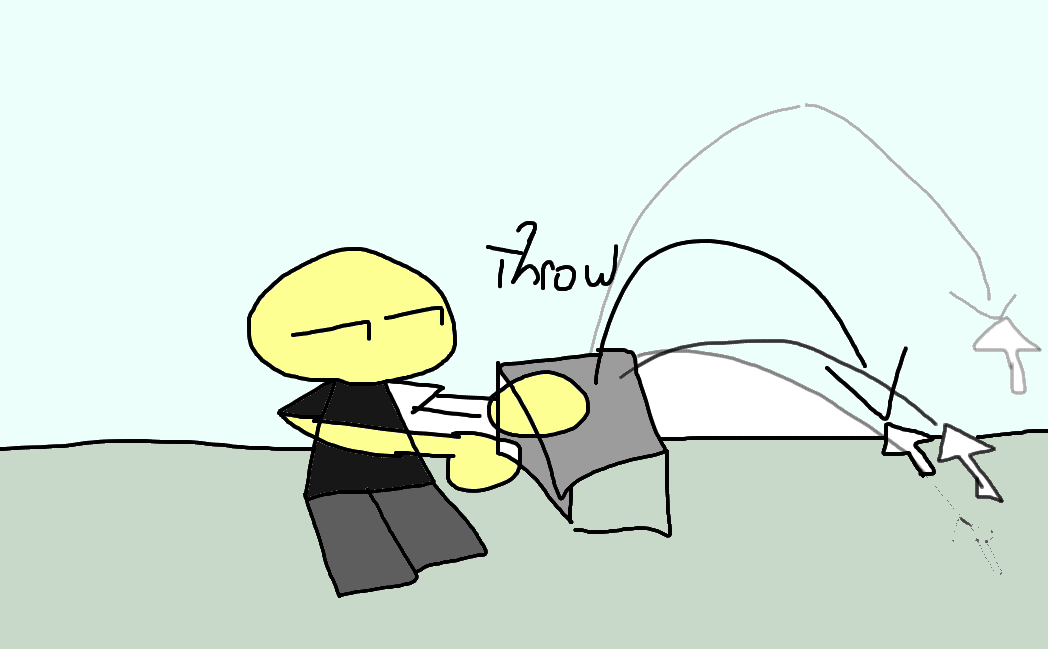# How should I go about making this?

Hello,

I’m wondering how should I make this. Basically, I’m making a game cald plox, where players race to smash as much bricks as they can while also trying to send the other player 5 feet under. I want to make it so that a player can get a brick (which I already have made). But I want the players to be able to throw the brick.

Here is an example of what I want.As you can see in the Image, xPlayer’s going to throw the object at xPos, which will be calculated with a formula with xMouse from xPlayer

Summary:
I want the brick in the players hands to go to a certain distance from the player’s Humanoid Root Part to the mouse position. How would I calculate this?

2 Likes

I’m assuming this is what you want:

``````-- Place this localscript in startercharacterscripts

local UIS = game:GetService("UserInputService") -- The service to detect inputs from the localplayer

local Part = Instance.new("Part") -- Instances a part
Part.Anchored = false -- Sets the part to not be anchored
Part.CanCollide = true -- Sets the part to have collision
Part.Position = script.Parent:WaitForChild("HumanoidRootPart").Position + Vector3.new(0,5,0) -- sets the parts position 5 studs above the player's position
Part.Parent = workspace -- Sets the part's parent to workspace, so it can be seen in game
return Part
end

UIS.InputBegan:Connect(function(input)
if input.UserInputType == Enum.UserInputType.MouseButton1 then -- Checks if the input is from the mouse button 1.
local MousePos = game.Players.LocalPlayer:GetMouse().Hit.Position -- Gets the mouse's position in the 3d world.
local Part = AddPart() -- Makes the variable part equal to the instanced part
Part:ApplyImpulse((MousePos - script.Parent:WaitForChild("HumanoidRootPart").Position) * 15) -- ApplyImpulse sends an impulse on a part. We set the impulse to the difference of MousePos and the player's position.
-- We set the impulse to that, because if we want to get from 1 to 4, we will need to get the offset by subtracting the 2 numbers. (Finish - start)
-- This makes sense because the inverse operation of addition is subtraction. We could count up from 1 to 4 and we would get 3, and if we did 4 - 0 we would get 4, but since our start is 1, we need the distance to start from 1, so therefore we would subtract 1 from 4.
end
end)
``````
3 Likes

Hey, instead of sending a script to me, please explain what it does, as it would be very helpful.

It does look like something I do want though, just want an explanation on how it works and how I can utilize it on my own.

2 Likes

3 Likes

Thanks!

2 Likes

Here is a helper function you can use to determine the angle:

``````function AngleOfReach(distance, altitude, velocity)
local gravity = workspace.Gravity
local theta = math.atan((velocity^2 + math.sqrt(velocity^4 -gravity*(gravity*distance^2 + 2*altitude*velocity^2)))/(gravity*distance))
if theta ~= theta then
theta = math.pi/4
end
return(theta)
end
``````

Next, assuming your “brick” part is a tool you can get the distance by this:

``````	local distance = (handlePos - mousePos).magnitude -- Get the distance between the handle and the mouse
``````
``````	local altitude = mousePosition.Y - tool.Handle.Position.Y
local angle = AngleOfReach(distance, altitude, config.GrenadeVelocity.Value) -- Calculate the angle
``````

Replace my config.GrenadeVelocity.Value with whatever you want. If you don’t know what number, you could just put like 150 and then change it higher or lower depending on what angle you are going for.

Then do some more calculations:

``````grenade.CFrame = tool.Handle.CFrame
``````

Again, replace all the instances of the grenade with your specific tool. I used this code for a grenade tool I made to make it throw.

here is a Roblox file with all this code in action:

3 Likes

I’ll try the script out in a second, but the only thing I don’t understand is why you decided to multiply the magnitude by 15.

2 Likes

Why should I look out for the angle of the part?.

2 Likes

I multiplied it because the impulse itself, did not have enough power to go to its desired position.

3 Likes

Does the value of `15` matter?

2 Likes

In that script, changing the 15 to be higher should make it go farther/faster

2 Likes

Multiplying the difference by the player’s assemblymass could achieve the same affect.

2 Likes

Could you show me an example of that?

2 Likes

Change the ApplyImpulse line to this.

``````Part:ApplyImpulse((MousePos - script.Parent:WaitForChild("HumanoidRootPart").Position) * script.Parent:WaitForChild("HumanoidRootPart").AssemblyMass)
``````
2 Likes

What’s AssemblyMass?

2 Likes
3 Likes

Ok, Thanks!

2 Likes

Actually, you would multiply it by the part’s assembly mass, because mass can affect the strength of the impulse.

`````` Part:ApplyImpulse((MousePos - script.Parent:WaitForChild("HumanoidRootPart").Position) * Part.AssemblyMass)
``````
3 Likes

Remade the script so it could be the same regardless of size:

``````local UIS = game:GetService("UserInputService")

local Part = workspace.Part:Clone()
if tonumber(script.Parent.Text) then
Part.Size = Vector3.new(script.Parent.Text,script.Parent.Text,script.Parent.Text)
end
Part.Anchored = false
Part.CanCollide = true
Part.Position = game.Players.LocalPlayer.Character:WaitForChild("HumanoidRootPart").Position + Vector3.new(0,5,0)
Part.Parent = workspace
return Part
end

UIS.InputBegan:Connect(function(input)
if input.UserInputType == Enum.UserInputType.MouseButton1 then
local MousePos = game.Players.LocalPlayer:GetMouse().Hit.Position